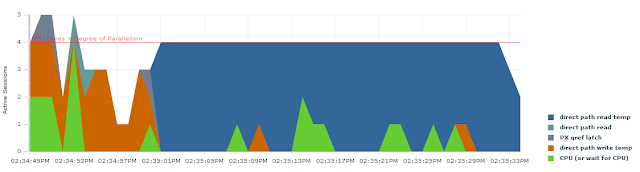## Sunday, November 17, 2013

Calculating number of unique values using Oracle distinct for big data sets has two major problems:
• It may require lots of memory for sort/hash group by.
• It is very difficult to refresh distinct numbers incrementally meaning every time you append some new data you generally have to perform distinct calculations from scratch.
Oracle introduced approximate NDV algorithm in 11G to solve the above problems but it's practical application is pretty much limited to stats gathering at the moment.

Places like Facebook and Google solve the above problems by using a very interesting algorithm called HyperLogLog. There is a pretty good description of how it works but, in a nutshell, it relies on counting the number of trailing (or leading) zeroes in the binary stream and using that information to estimate number of unique values with a very high degree of accuracy.

HyperLogLog is widely used due to the following properties:
• Extremely low memory footprint -- 1KB of memory is enough to estimate the number of unique values with a very high degree of precision across billions of rows.
• It can be used to refresh the numbers incrementally by calculating HyperLogLog for the new data set and then combining it with the HyperLogLog for the existing one.
• It is very parallel friendly. Each piece of data can be independently computed before being combined for the final result -- essentially utilizing the same mechanism as would be used for incremental refresh.
After reading a couple of HyperLogLog papers I became fascinated by it. The elegance of the math behind it is simply brilliant. So I became naturally curious to try it out in Oracle.

Of course the simplest way was to implement it as a user-defined aggregate, which I did. Unfortunately the performance of that solution left a lot to be desired due to a simple fact that there is quite a bit of overhead in executing a user-defined aggregate function for each row in the data set. Based on that I don't think there is a lot of practical applications for HyperLogLog in this form apart from doing a technology demo.

However, it's quite simple to compute most of the HyperLogLog directly inside the SQL statement itself and, as expected, that provides a much better performance. So here is a quick example which demonstrates HyperLogLog in action.

Fist I will create a test table:
```SQL> create table z_hll_test as
2   select dbms_random.string('x', 4)||rpad('x', 500, 'x') n
3    from dual
4    connect by level <= 1000000;

Table created```
Next I will set my pga_aggregate_target=64m to simulate a situation where distinct will have to do a lot of spilling to disk due to large amounts of data being processed as I don't want to spent a lot of time generating hundreds of billions of rows instead.

First let's run Oracle's distinct:
```SQL> set timing on
SQL> select /*+ parallel(z 4) */ count(distinct n) from z_hll_test z;
753521

Elapsed: 00:00:46.02```
As you may figure out, most of the 46 seconds were spent spilling to temp:Let's see what happens if we use HyperLogLog instead. First I will create a function to calculate the number of trailing zeroes we have in a number:
```create or replace function num_zeroes(
p_n binary_integer
) return binary_integer deterministic is
l_t binary_integer;
l_b binary_integer;
begin
--assume 32-bit hash value, 10-bits for bucket
if (p_n = 0) then return 22; end if;

l_t := 1;
l_b := 0;

while ( bitand(p_n,l_t) = 0 )
loop
l_t := l_t*2;
l_b := l_b+1;
end loop;

return l_b;

end num_zeroes;```
Now we can use this function to compute the HyperLogLog value directly in a SQL statement:
```SQL> select
case
2    3                when hll <= 2560 and zeroes > 0 then round(1024*ln(1024*1/zeroes))
when hll >  1/30 * power(2,32) then round(-power(2,32) * ln(1 - hll/power(2,32)))
4    5                else round(hll)
6     end num_distinct
7  from (
8  select 0.72054 * (1048576/(current_sum+zeroes)) hll, zeroes
9  from (
10     select sum(1/power(2, val)) current_sum, (1024-count(*)) zeroes
11     from (
12             select /*+ parallel(z 4) */
13                             mod(ora_hash(n), 1024) bucket,
14                             max(num_zeroes(trunc(ora_hash(n)/1024)))+1 val
15                     from z_hll_test z
16                     group by mod(ora_hash(n), 1024)
17             )
18     )
19  );
738105

Elapsed: 00:00:02.08
```
The SQL might look a bit complicated but it's pretty straightforward once you realize how HyperLogLog works (see the link I provided above). For you see, the performance is 22x faster because the entire thing was able to compute in-memory (there are only 1024 distinct values left after the group by as far as Oracle is concerned) and result is within 2% of the precise distinct count -- very impressive!

The algoirithm can have even more potential in the Exadata environments, if we imagine for a second that Oracle gives us a native implementation which is off-loaded, because each cell can independently compute it's part of the data and then simply sent the results out for final merge. Indeed, this is how places like Facebook and Google scale it across lots of small servers.

1.what does the "0.72054" mean ? A magic Number?

2.The algorithm has a certain "bias" depending on how many buckets you have. This number is used to correct it and is calculated using a formula (0.7213 / (1 + 1.079 / number_of_buckets)) = (0.7213 / (1 + 1.079 / 1024)) = 0.72054

3.Anonymous9:28 a.m.

How come the number of distinct values is 753521 from the first query and 738105 from the second ?

1.HLL is not precise. It's an estimation. count(distinct) gives you the exact value.